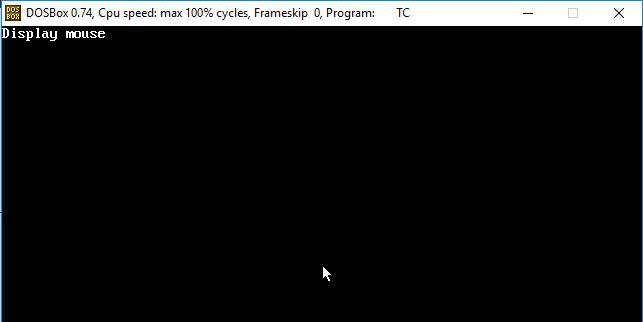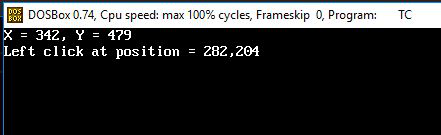GFG App
Open AppBrowser
Continue

# Use cases of mouse programming in C/C++

In this article, we will discuss some use cases of Mouse Programming:

### Display Mouse Pointer in graphics mode:

For displaying the Mouse Pointer, first enable the graphic mode using initgraph() function, if it is successfully initialized than it calls initMouse() function to check mouse is available or not, If initMouse() function returns not equal 0 means it will return ffffh then it calls the showMouse() function and display the cursor on the console window in graphic mode. Below are the functions used:

• initMouse(): use to initialized mouse.
• showMouse(): shows the mouse pointer on the output screen.

Below is the program for the same:

## C

 `// C program to display Mouse ` `// pointer in Graphics Mode ` `#include ` `#include ` `#include ` `#include ` `union` `REGS in, out; ` ` `  `// Function to display the mouse ` `// pointer ` `void` `showMouse() ` `{ ` `    ``// Set service AX = 1 for ` `    ``// displaying mouse ` `    ``in.x.ax = 1; ` `    ``int86(0X33, &in, &out); ` `} ` ` `  `// Function to initialize the mouse ` `// pointer ` `int` `initMouse() ` `{ ` `    ``// Set service AX = 0 for ` `    ``// detecting mouse ` `    ``in.x.ax = 0; ` `    ``int86(0x33, &in, &out); ` `    ``return` `out.x.ax; ` `} ` ` `  `// Driver Code ` `void` `main() ` `{ ` `    ``int` `status, gd = DETECT, gm; ` `    ``clrscr(); ` ` `  `    ``initgraph(&gd, &gm, ` `              ``"C:\\TURBOC3\\BGI"``); ` ` `  `    ``// Get the status of mouse pointer ` `    ``status = initMouse(); ` ` `  `    ``// Check mouse is available or not ` `    ``if` `(status == 0) ` `        ``printf``(``"Mouse support not"` `               ``" available.\n"``); ` `    ``else` `{ ` `        ``printf``(``"Display mouse"``); ` `        ``showMouse(); ` `    ``} ` ` `  `    ``getch(); ` ` `  `    ``// Close the graphics ` `    ``closegraph(); ` `}`

## C++

 `// C++ program to display Mouse ` `// pointer in Graphics Mode ` `#include ` `#include ` `#include ` `#include ` `#include ` `using` `namespace` `std; ` `union` `REGS in, out; ` ` `  `// Function to display the mouse ` `// pointer ` `void` `showMouse() ` `{ ` `    ``// Set service AX = 1 for ` `    ``// displaying mouse ` `    ``in.x.ax = 1; ` `    ``int86(0X33, &in, &out); ` `} ` ` `  `// Function to initialize the mouse ` `// pointer ` `int` `initMouse() ` `{ ` `    ``// Set service AX = 0 for ` `    ``// detecting mouse ` `    ``in.x.ax = 0; ` `    ``int86(0x33, &in, &out); ` ` `  `    ``return` `out.x.ax; ` `} ` ` `  `// Driver Code ` `void` `main() ` `{ ` `    ``int` `status, gd = DETECT, gm; ` `    ``clrscr(); ` ` `  `    ``initgraph(&gd, &gm, ``"C:\\TURBOC3\\BGI"``); ` ` `  `    ``// Get the status of mouse pointer ` `    ``status = initMouse(); ` ` `  `    ``// Check if mouse is available or not ` `    ``if` `(status == 0) ` `        ``cout << ``"Mouse support not"` `             ``<< ``" available.\n"``; ` `    ``else` `{ ` `        ``cout << ``"Display mouse"``; ` `        ``showMouse(); ` `    ``} ` ` `  `    ``getch(); ` ` `  `    ``// Close the graphics ` `    ``closegraph(); ` `}`

Output:### Hide Mouse Pointer:

For hiding the mouse pointer, first check the status of mouse if it is available than simply control will enter in for loop make delay of 500 millisecond  then the mouse will appear, then disappear after 500 milliseconds and will show again after 500 millisecond. This sequence will continue until the loop ends. The delay function has been used to show you the impact of  hidemouse() function .

• initmouse(): use to initialized mouse.
• showmouse(): shows the mouse pointer on the output screen.
• hidemouse(): hides the mouse pointer

Below is the program for the same:

## C

 `// C program for hiding Mouse Pointer ` `#include ` `#include ` `#include ` ` `  `union` `REGS in, out; ` ` `  `// Function to initialize the mouse ` `// pointer using graphics ` `int` `initMouse() ` `{ ` `    ``in.x.ax = 0; ` `    ``int86(0X33, &in, &out); ` ` `  `    ``return` `out.x.ax; ` `} ` ` `  `// Function to display the mouse ` `// pointer using graphics ` `void` `showMouse() ` `{ ` `    ``in.x.ax = 1; ` `    ``int86(0X33, &in, &out); ` `} ` ` `  `// Function to hide the mouse ` `// pointer using graphics ` `void` `hideMouse() ` `{ ` `    ``// Set AX=2 to hide mouse ` `    ``in.x.ax = 2; ` `    ``int86(0X33, &in, &out); ` `} ` ` `  `// Driver Code ` `void` `main() ` `{ ` `    ``int` `status, i, gd = DETECT, gm; ` ` `  `    ``// Initialize graphics ` `    ``initgraph(&gd, &gm, ``"C:\\TURBOC3\\BGI"``); ` ` `  `    ``// Get the status of the mouse ` `    ``status = initMouse(); ` ` `  `    ``// Check if mouse is available or not ` `    ``if` `(status == 0) ` `        ``printf``(``"Mouse support "` `               ``"not available.\n"``); ` `    ``else` `{ ` `        ``for` `(i = 0; i <= 10; i++) { ` ` `  `            ``// Pause execution for .5 sec ` `            ``delay(500); ` `            ``showMouse(); ` `            ``delay(500); ` `            ``hideMouse(); ` `        ``} ` `    ``} ` ` `  `    ``getch(); ` ` `  `    ``// Close the graphics ` `    ``closegraph(); ` `}`

## C++

 `// C++ program for hiding Mouse Pointer ` `#include ` `#include ` `#include ` `#include ` `using` `namespace` `std; ` ` `  `union` `REGS in, out; ` ` `  `// Function to initialize the mouse ` `// pointer using graphics ` `int` `initMouse() ` `{ ` `    ``in.x.ax = 0; ` `    ``int86(0X33, &in, &out); ` ` `  `    ``return` `out.x.ax; ` `} ` ` `  `// Function to display the mouse ` `// pointer using graphics ` `void` `showMouse() ` `{ ` `    ``in.x.ax = 1; ` `    ``int86(0X33, &in, &out); ` `} ` ` `  `// Function to hide the mouse ` `// pointer using graphics ` `void` `hideMouse() ` `{ ` `    ``// Set AX=2 to hide mouse ` `    ``in.x.ax = 2; ` `    ``int86(0X33, &in, &out); ` `} ` ` `  `// Driver Code ` `void` `main() ` `{ ` `    ``int` `status, i, gd = DETECT, gm; ` ` `  `    ``// Initialize graphics ` `    ``initgraph(&gd, &gm, ``"C:\\TURBOC3\\BGI"``); ` ` `  `    ``// Get the status of the mouse ` `    ``status = initMouse(); ` ` `  `    ``// Check if mouse is available or not ` `    ``if` `(status == 0) ` `        ``cout << ``"Mouse support "` `             ``<< ``"not available.\n"``; ` `    ``else` `{ ` `        ``for` `(i = 0; i <= 10; i++) { ` ` `  `            ``// Pause execution for .5 sec ` `            ``delay(500); ` `            ``showMouse(); ` `            ``delay(500); ` `            ``hideMouse(); ` `        ``} ` `    ``} ` ` `  `    ``getch(); ` ` `  `    ``// Close the graphics ` `    ``closegraph(); ` `}`

Output:

### Determine the current mouse position and button clicked:

In this program, the current location of the mouse is printed as well as which key of the mouse is being clicked at which location using the below functions:

• initmouse(): use to initialized mouse.
• showmouse(): shows the mouse cursor on the output screen.
• getmouseposition(): fetches the mouse coordinate & which key is being pressed in BX, CX, DX register respectively.
• If BX = 1 left key clicked
• If BX = 2 Right key clicked.
• If BX = 3 Center Button is Pressed
• CX = Mouse X Coordinate
• DX = Mouse Y Coordinate

Below is the program for the same:

## C

 `// C program to determine the current ` `// mouse position and button clicked ` `#include ` `#include ` `#include ` `#include ` `union` `REGS in, out; ` ` `  `// Function to initialize the mouse ` `// pointer using graphics ` `int` `initMouse() ` `{ ` `    ``in.x.ax = 0; ` `    ``int86(0X33, &in, &out); ` ` `  `    ``return` `out.x.ax; ` `} ` ` `  `// Function to display the mouse ` `// pointer using graphics ` `void` `showMouse() ` `{ ` `    ``in.x.ax = 1; ` `    ``int86(0X33, &in, &out); ` `} ` ` `  `// Function to get the current clicked ` `// mouse position on the screen ` `void` `getMousePosition(``int``* click, ` `                      ``int``* x, ``int``* y) ` `{ ` `    ``in.x.ax = 3; ` `    ``int86(0X33, &in, &out); ` ` `  `    ``// Get the coordinates ` `    ``*click = out.x.bx; ` ` `  `    ``// Update the x and y coordinates ` `    ``*x = out.x.cx; ` `    ``*y = out.x.dx; ` `} ` ` `  `// Driver Code ` `void` `main() ` `{ ` `    ``int` `status, i, gd = DETECT, gm; ` ` `  `    ``// Initialize graphics ` `    ``initgraph(&gd, &gm, ``"C:\\TURBOC3\\BGI"``); ` ` `  `    ``// Get the status of the mouse ` `    ``status = initMouse(); ` ` `  `    ``// Check if mouse is available or not ` `    ``if` `(status == 0) ` `        ``printf``(``"Mouse support "` `               ``"not available.\n"``); ` `    ``else` `{ ` ` `  `        ``showMouse(); ` ` `  `        ``// Get the current mouse position ` `        ``getMousePosition(&click, &x, &y); ` ` `  `        ``while` `(!kbhit()) { ` ` `  `            ``getMousePosition(&click, &x, &y); ` `            ``gotoxy(1, 1); ` `            ``printf``(``"X = %d, Y = %d"``, x, y); ` ` `  `            ``if` `(click == 1) ` `                ``printf``(``"\nLeft click at "` `                       ``"position = %d, %d\t"``, ` `                       ``x, y); ` `            ``if` `(click == 2) ` `                ``printf``(``"\nRight click at "` `                       ``"position = %d, %d\t"``, ` `                       ``x, y); ` `        ``} ` `    ``} ` ` `  `    ``getch(); ` ` `  `    ``// Close the graphics ` `    ``closegraph(); ` `}`

## C++

 `// C program to determine the current ` `// mouse position and button clicked ` `#include ` `#include ` `#include ` `#include ` `#include ` `using` `namespace` `std; ` `union` `REGS in, out; ` ` `  `// Function to initialize the mouse ` `// pointer using graphics ` `int` `initMouse() ` `{ ` `    ``in.x.ax = 0; ` `    ``int86(0X33, &in, &out); ` ` `  `    ``return` `out.x.ax; ` `} ` ` `  `// Function to display the mouse ` `// pointer using graphics ` `void` `showMouse() ` `{ ` `    ``in.x.ax = 1; ` `    ``int86(0X33, &in, &out); ` `} ` ` `  `// Function to get the current clicked ` `// mouse position on the screen ` `void` `getMousePosition(``int``* click, ` `                      ``int``* x, ``int``* y) ` `{ ` `    ``in.x.ax = 3; ` `    ``int86(0X33, &in, &out); ` ` `  `    ``// Get the coordinates ` `    ``*click = out.x.bx; ` ` `  `    ``// Update the x and y coordinates ` `    ``*x = out.x.cx; ` `    ``*y = out.x.dx; ` `} ` ` `  `// Driver Code ` `void` `main() ` `{ ` `    ``int` `status, i, gd = DETECT, gm; ` ` `  `    ``// Initialize graphics ` `    ``initgraph(&gd, &gm, ``"C:\\TURBOC3\\BGI"``); ` ` `  `    ``// Get the status of the mouse ` `    ``status = initMouse(); ` ` `  `    ``// Check if mouse is available or not ` `    ``if` `(status == 0) ` `        ``printf``(``"Mouse support "` `               ``"not available.\n"``); ` `    ``else` `{ ` ` `  `        ``showMouse(); ` ` `  `        ``// Get the current mouse position ` `        ``getMousePosition(&click, &x, &y); ` ` `  `        ``while` `(!kbhit()) { ` ` `  `            ``getMousePosition(&click, &x, &y); ` `            ``gotoxy(1, 1); ` `            ``printf``(``"X = %d, Y = %d"``, x, y); ` ` `  `            ``if` `(click == 1) ` `                ``cout << ``"\nLeft click at "` `                     ``<< ``"position = "` `                     ``<< x << ``", "` `<< y; ` ` `  `            ``if` `(click == 2) ` `                ``cout << ``"\nRight click at "` `                     ``<< ``"position = "` `                     ``<< x << ``", "` `<< y; ` `        ``} ` `    ``} ` ` `  `    ``getch(); ` ` `  `    ``// Close the graphics ` `    ``closegraph(); ` `}`

Output:My Personal Notes arrow_drop_up